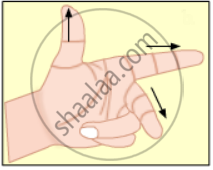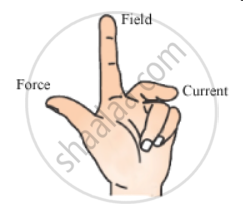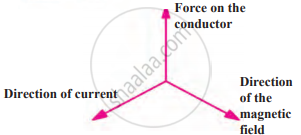# Name the Following Diagrams and Explain the Concept Behind Them. - Science and Technology 1

Diagram

Name the following diagram and explain the concept behind them.#### Solution

It represents Fleming's left hand rule used for finding the direction of magnetic force when a current carrying conductor is placed in a magnetic field.This rule states that if you stretch the thumb, index finger, and middle finger of your left hand such that they are mutually perpendicular to each other, then your index finger represents the direction of the field, the middle finger represents the direction of the current, and the thumb represents the direction of the force experienced by the conductor.

Concept: Force on a Current Carrying Conductor in a Magnetic Field
Is there an error in this question or solution?